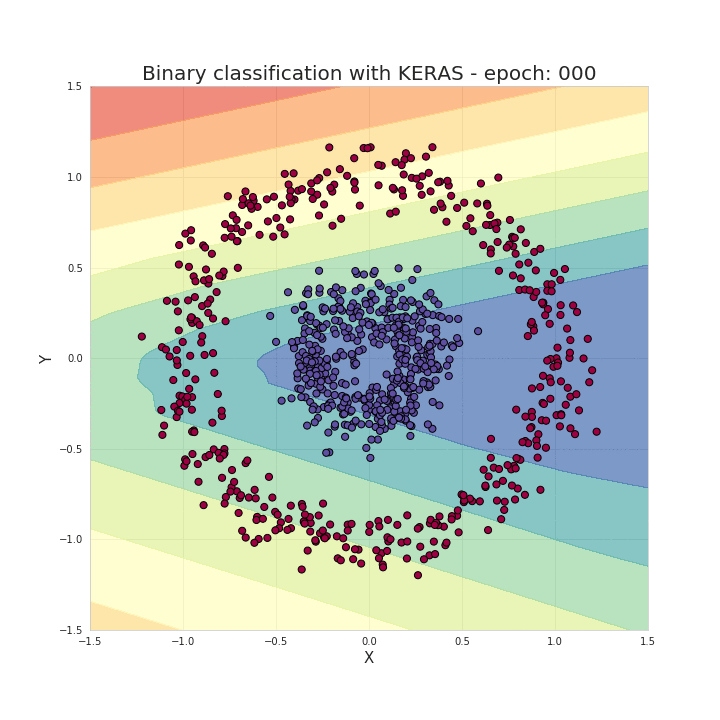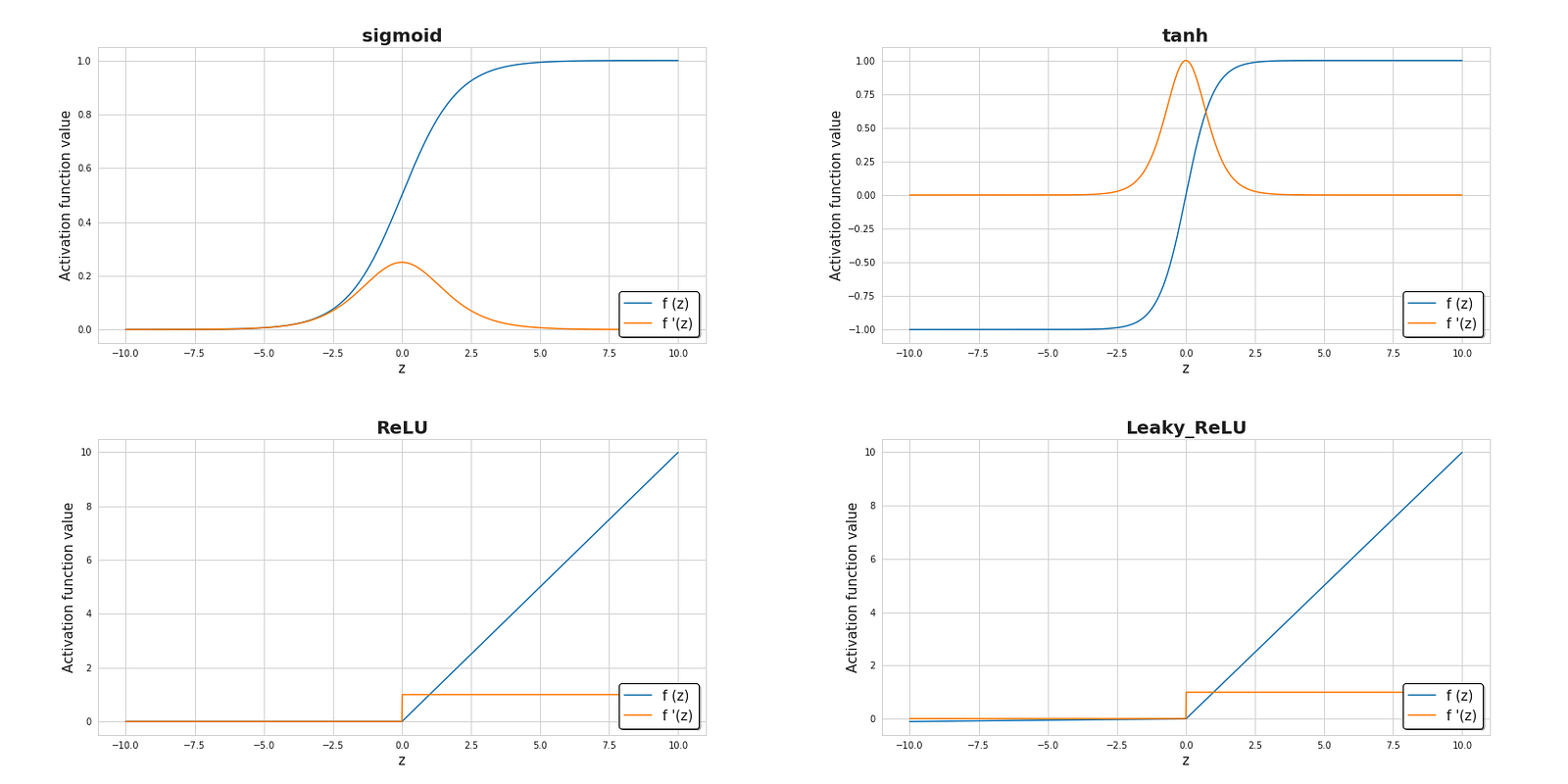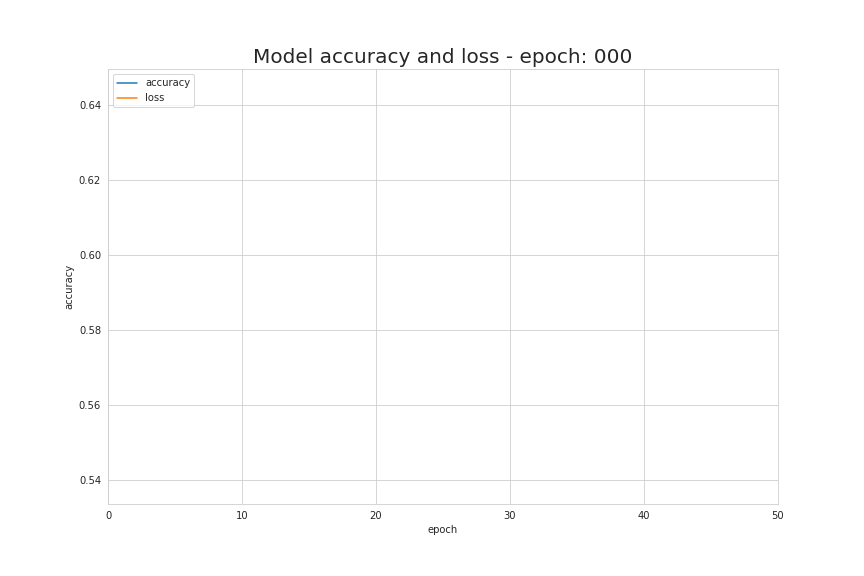towardsdatascience.com Go back Open original

# Deep Dive into Math Behind Deep Networks

By Piotr Skalski

Nowadays, having at our disposal many high-level, specialized libraries and frameworks such as Keras, TensorFlow or PyTorch, we do not need to constantly worry about the size of our weights matrices or remembers formula for the derivative of activation function we decided to use. Often all we need to create a neural network, even one with a very complicated structure, is a few imports and a few lines of code. This saves us hours of searching for bugs and streamlines our work. However, the knowledge of what is happening inside the neural network helps a lot with tasks like architecture selection, hyperparameters tuning or optimisation.

Note: Thanks to the Jung Yi Lin courtesy, you can also read this article in Chinese. You can also check out the Portuguese version of the text thanks to Davi Candido help. The source code used to create visualizations that were used in this article is available on my GitHub.

To understand more about how neural networks work, I decided to spend some time in this summer and take a look at the mathematics that hides under the surface. I also decided to write an article, a bit for myself — to organize newly learned information, a bit for others — to help them understand these sometimes difficult concepts. I will try to be as gentle as possible for those who feel less comfortable with algebra and differential calculus, but as the title suggests, it will be an article with a lot of math.

As an example, we will solve the problem of the binary classification of the data set, which is presented in the Figure 1. above. Points belonging to two classes form circles — this arrangement is inconvenient for many traditional ML algorithms, but a small neural network should work just fine. In order to tackle this problem, we will use a NN with the structure shown in Figure 2. — five fully connected layers, with different numbers of units. For hidden layers we will use the ReLU as the activation function, and Sigmoid for the output layer. It is a quite simple architecture, but complicated enough to be a useful example for our deliberations.

First, I will present a solution using one of the most popular machine-learning libraries — KERAS.

Aaaaand that’s it. As I mentioned in the introduction, a few imports and a few lines of code are enough to create and train a model that is then able to classify the entries from our test set with almost 100% accuracy. Our task boils down to providing hyperparameters (number of layers, the number of neurons in the layer, activation functions or the number of epochs) in accordance with the selected architecture. Let us now look at what happened behind the scenes. Oh… And a cool visualization I created during the learning process, which hopefully keep you from falling asleep.Figure 3. Visualization of areas qualified for appropriate classes during training

Let us start by answering this key question: What is a neural network? It is a biologically-inspired method of building computer programs that are able to learn and independently find connections in data. As Figure 2. shows, nets are a collection of software ‘neurons’ arranged in layers, connected together in a way that allows communication.

Each neuron receives a set of x-values (numbered from 1 to n) as an input and compute the predicted y-hat value. Vector x actually contains the values of the features in one of m examples from the training set. What is more each of units has its own set of parameters, usually referred to as w (column vector of weights) and b (bias) which changes during the learning process. In each iteration, the neuron calculates a weighted average of the values of the vector x, based on its current weight vector w and adds bias. Finally, the result of this calculation is passed through a non-linear activation function g. I will mention a bit about the most popular activation functions in the following part of the article.

Now let’s zoom out a little and consider how calculations are performed for a whole layer of the neural network. We will use our knowledge of what is happening inside a single unit and vectorize across full layer to combine those calculations in into matrix equations. To unify the notation, the equations will be written for the selected layer [l]. By the way, subscript i mark the index of a neuron in that layer.

One more important remark: When we wrote the equations for a single unit, we used x and y-hat, which were respectively the column vector of features and the predicted value. When switching to the general notation for layer, we use the vector a — meaning the activation of the corresponding layer. The x vector is therefore the activation for layer 0 — input layer. Each neuron in the layer performs a similar calculation according to the following equations:

For the sake of clarity, let’s write down the equations for example for layer 2:

As you can see, for each of the layers we have to perform a number of very similar operations. Using for-loop for this purpose is not very efficient, so to speed up the calculation we will use vectorization. First of all, by stacking together horizontal vectors of weights w (transposed) we will build matrix W. Similarly, we will stack together bias of each neuron in the layer creating vertical vector b. Now there is nothing to stop us from building a single matrix equations that allows us to perform calculations for all the neurons of the layer at once. Let’s also write down the dimensions of the matrices and vectors we have used.

The equation that we have drawn up so far involves only one example. During the learning process of a neural network, you usually work with huge sets of data, up to millions of entries. The next step will therefore be vectorisation across multiple examples. Let’s assume that our data set has m entries with nx features each. First of all, we will put together the vertical vectors x, a, and z of each layer creating the X, A and Z matrices, respectively. Then we rewrite the previously laid-out equation, taking into account the newly created matrices.

Activation functions are one of the key elements of the neural network. Without them, our neural network would become a combination of linear functions, so it would be just a linear function itself. Our model would have limited expansiveness, no greater than logistic regression. The non-linearity element allows for greater flexibility and creation of complex functions during the learning process. The activation function also has a significant impact on the speed of learning, which is one of the main criteria for their selection. Figure 6 shows some of the commonly used activation functions. Currently, the most popular one for hidden layers is probably ReLU. We still sometimes use sigmoid, especially in the output layer, when we are dealing with a binary classification and we want the values returned from the model to be in the range from 0 to 1.Figure 6. Diagrams of the most popular activation functions together with their derivatives.

The basic source of information on the progress of the learning process is the value of the loss function. Generally speaking, the loss function is designed to show how far we are from the ‘ideal’ solution. In our case we used binary crossentropy, but depending on the problem we are dealing with different functions can be applied. The function used by us is described by the following formula, and the change of its value during the learning process is visualised in Figure 7. It shows how with each iteration the value of the loss function decreases and accuracy increases.Figure 7. Change of accuracy and loss values during learning process

The learning process is about changing the values of the W and b parameters so that the loss function is minimized. In order to achieve this goal, we will turn for help to calculus and use gradient descent method to find a function minimum. In each iteration we will calculate the values of the loss function partial derivatives with respect to each of the parameters of our neural network. For those who are less familiar with this type of calculations, I will just mention that the derivative has a fantastic ability to describe the slope of the function. Thanks to that we know how to manipulate variables in order to move downhill in the graph. Aiming to form an intuition about how the gradient descent works (and stop you from falling asleep once again) I prepared a small visualization. You can see how with each successive epoch we are heading towards the minimum. In our NN it works in the same way — the gradient calculated on each iteration shows us the direction in which we should move. The main difference is that in our exemplary neural network, we have many more parameters to manipulate. Exactly… How to calculate such complex derivatives?